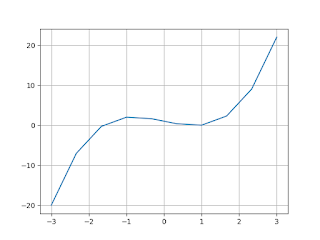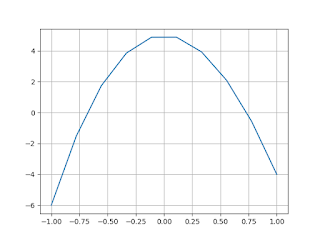# Program for Newton Raphson Method in Python

In this, first we compare this method with Bisection method. What are the major points in the both methods. Then we discuss about the Newton Raphson Method.

1. In the Bisection method, we were given a interval. Here we  need the initial estimated value of the root.

2. While the previous two methods are guaranteed to converge, Newton Raphson may not converge in some cases.

3. The Newton Raphson method requires a derivative. Some functions may be difficult. It is impossible to separate.

4. For many problems, the Newton Raphson method converge faster than the two methods above.

Also, it can locate roots repeatedly because it does not clearly see changes in the sign of f (x) explicitly.

### Newton Raphson Method

#### Steps:

• Input: initial x, func (x), derivfunc (x)
• Output: Root of Func ()
• Calculate the values   of funk (x) and derivfunc (x) for a given initial x
• Calculate H: h = func (x) / derivFunc (x) If h is greater than the allowable error ε
• h = func (x) / derivFunc (x)
• x = x - h
Now take a look at different examples used to find the root of the given function.

#### Example 1: Finding the root of equation x**3-x**2+2

Python Code:
`def func(x):    y = x**3-x**2+2    return ydef derifunc(x):    y = 3*x*x-2*x    return yc = -20def newtonraphson(x):    h = func(x)/derifunc(x)    while abs(h)>=0.001:        h = func(x)/derifunc(x)        x = x-h    print("the root of equation is: %4f"%x)newtonraphson(c)`
`Output:`
```the root of equation is: -1.000000
```

#### Example 2: Finding the root of equation 3x-cosx-1

`Python Code`
`from pylab import *def f(x):    y = 3*x-np.cos(x)-1    return yx = linspace(-3,3,10)def derivative (x):    h = 0.000001    derivative = (f(x+h)-f(x))/h    return derivativedef newtonraphson(x):    return (x-(f(x)/derivative(x)))def iterate(p,n):    x = p    for i in range(n):        x = newtonraphson(x)    return xprint("root of equation is:",iterate(1,3))plot(x,f(x))grid(True)show()Output:`
```root of equation is: 0.6071016481468803
```#### Example 3: Finding the root of equation x3-2x+1

`Python Code:`
`from pylab import *def f(x):    y = x**3-2*x+1    return yx = linspace(-3,3,10)def derivative (x):    h = 0.000001    derivative = (f(x+h)-f(x))/h    return derivativedef newtonraphson(x):    return (x-(f(x)/derivative(x)))def iterate(p,n):    x = p    for i in range(n):        x = newtonraphson(x)    return xprint("root of equation is:",iterate(1,3))plot(x,f(x))grid(True)show()`
Output:#### Example 4: Find the root of equation x3-10x2+5

Python Code:
`from pylab import *def f(x):    y = x*x*x-10*x*x+5    return yx = linspace(-1,1,10)def derivative (x):    h = 0.0001    derivative = (f(x+h)-f(x))/h    return derivativedef newtonraphson(x):    return (x-(f(x)/derivative(x)))def iterate(p,n):    x = p    for i in range(n):        x = newtonraphson(x)    return xprint("root of equation is:",iterate(1,3))plot(x,f(x))grid(True)show()`
`Output:`
```root of equation is: 0.7346036997195792
```